# java Lambda表达式

## Lambda表达式

Lambda 表达式是 JDK8 的一个新特性，可以取代大部分的匿名内部类，写出更优雅的 Java 代码，尤其在集合的遍历和其他集合操作中，可以极大地优化代码结构。

JDK 也提供了大量的内置函数式接口供我们使用，使得 Lambda 表达式的运用更加方便、高效。

## 快速上手

``````    public static void main(String[] args) throws Exception {
Runnable runnable = new Runnable() {
@Override
public void run() {
System.out.println("hello world");
}
};
runnable.run();
}
``````

Lambda表达式:

``````    public static void main(String[] args) throws Exception {
Runnable runnable = ()->System.out.println("hello world");
runnable.run();
}
``````

## 语法格式

Lambda通过 3个部分的语法结构来实现:

``````(x) ->  {}
``````
• (x) 第一部分为参数,表示需要声明定义传入的参数,类似于方法的形参声明,如果没有参数可以省略
• -> Lambda表达式 操作符
• {} 语句块,表示具体实现的方法代码,如果只有一行可以省略{}

### 语法格式一,有参数,有返回值,多行代码

``````    public static void main(String[] args) throws Exception {
Comparator<Integer> comparator = (Integer x,Integer y) -> {
System.out.println("函数式接口");
return Integer.compare(x, y);
};
System.out.println(comparator.compare(1,2));
}
``````

### 语法格式二,无参数,无返回值,一行语句

``````    public static void main(String[] args) throws Exception {
Runnable runnable = ()->System.out.println("hello world");
runnable.run();
}
``````

### 语法格式三,有一个参数

``````    public static void main(String[] args) throws Exception {
Consumer<String> func = s -> System.out.println(s);
func.accept("hello world");
}
``````

### 语法格式四,一行语句有返回值

``````    public static void main(String[] args) throws Exception {
Comparator<Integer> comparator = (x, y) ->  Integer.compare(x, y);
System.out.println(comparator.compare(1,1));
}
``````

### 语法格式五,可以省略形参类型,会自己实现类型推断

``````    public static void main(String[] args) throws Exception {
Comparator<Integer> comparator = (x, y) -> {
System.out.println("函数式接口");
return Integer.compare(x, y);
};
System.out.println(comparator.compare(1,2));
}
``````

##### 热门推荐
•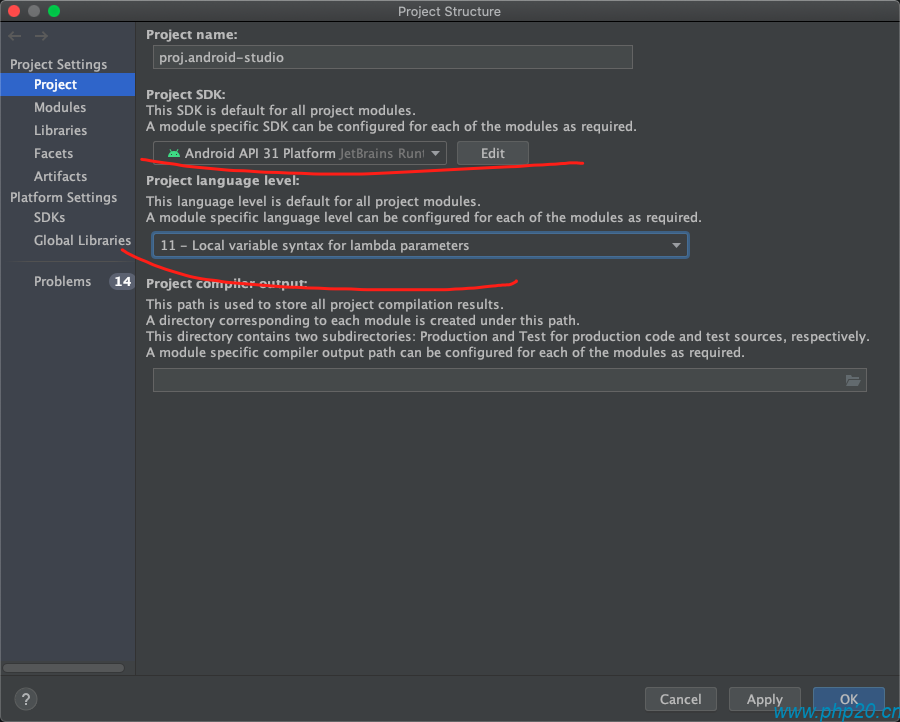浏览(2,242)
•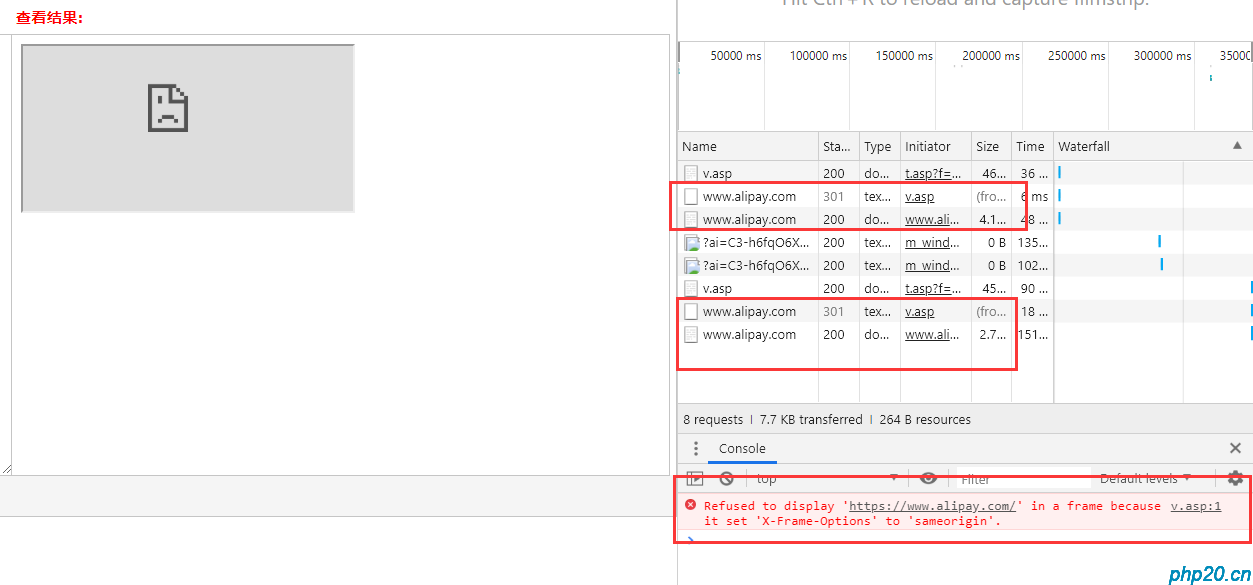浏览(1,298)
••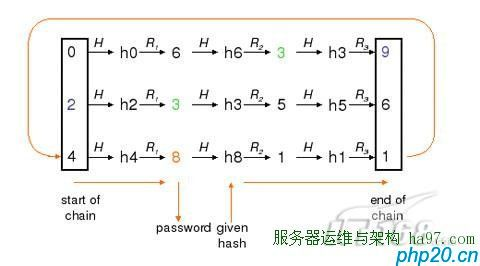•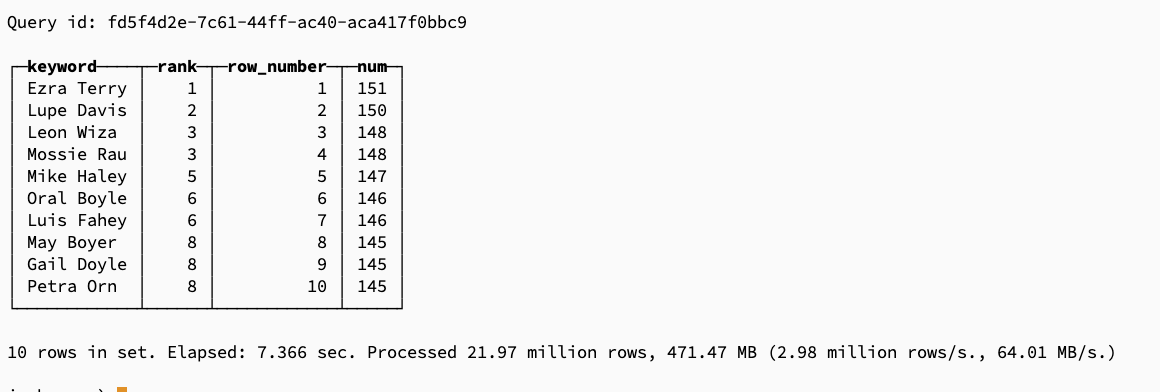浏览(1,011)
•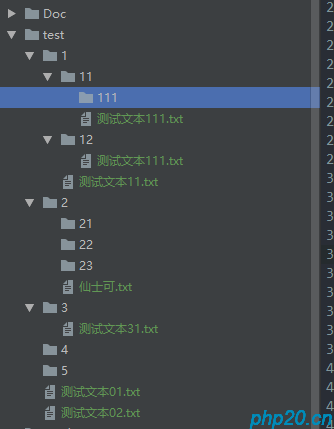浏览(964)
•浏览(915)
•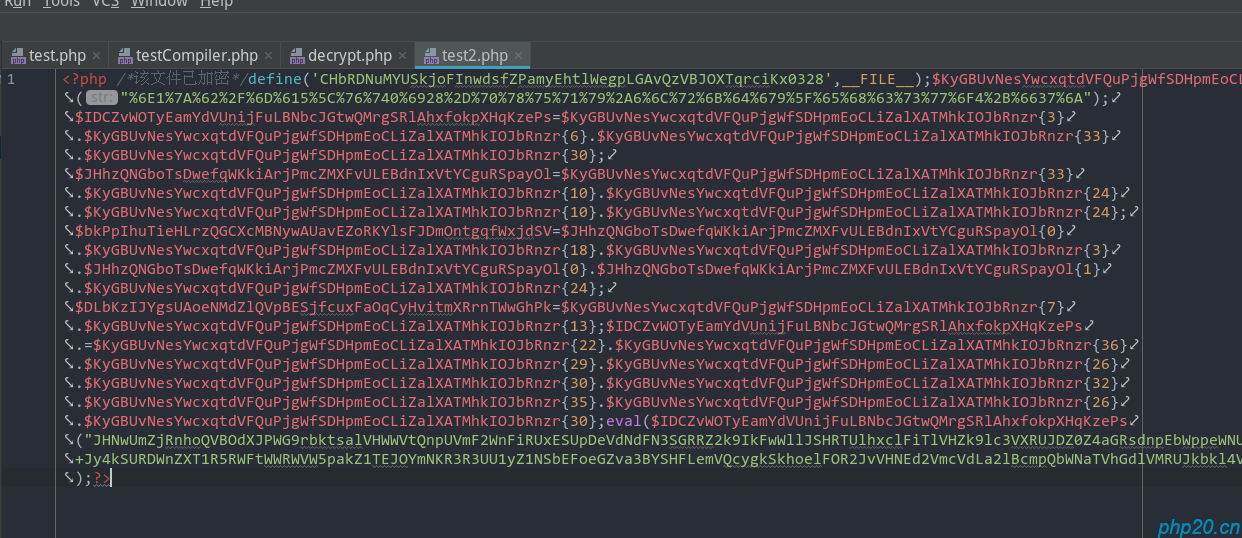浏览(864)
•浏览(842)
•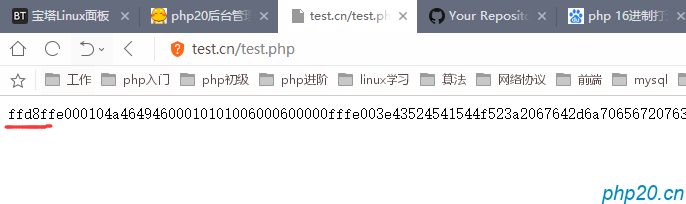浏览(735)